# PageRank算法原理与实现

+关注继续查看

## 1 PageRank

### 1.1 简介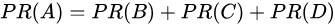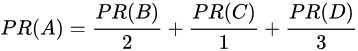### 1.2 公式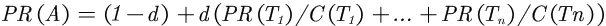• PR(A) 是页面A的PR值
• PR(Ti)是页面Ti的PR值，在这里，页面Ti是指向A的所有页面中的某个页面
• C(Ti)是页面Ti的出度，也就是Ti指向其他页面的边的个数
• d 为阻尼系数，其意义是，在任意时刻，用户到达某页面后并继续向后浏览的概率，该数值是根据上网者使用浏览器书签的平均频率估算而得，通常d=0.85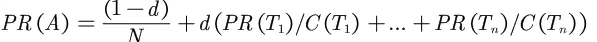N为页面的总数

### 1.3 具体实例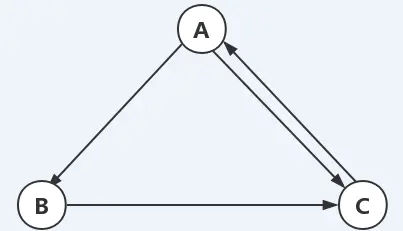• 页面A的PR值计算如下：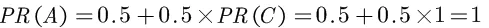• 页面B的PR值计算如下：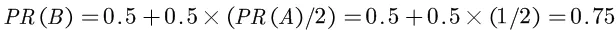• 页面C的PR值计算如下：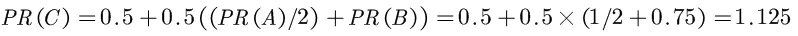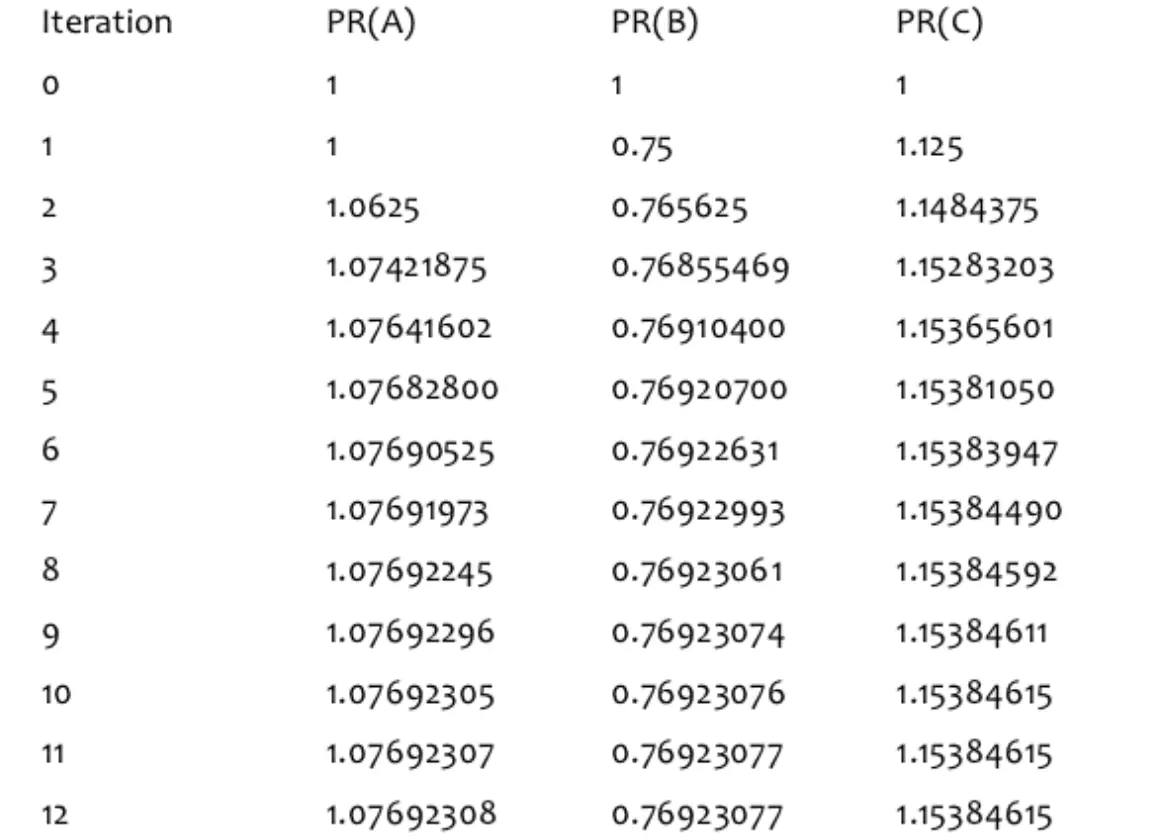## 2 代码实现

import numpy as np
from scipy.sparse import csc_matrix
def pageRank(G, s=.85, maxerr=.0001):
"""
Computes the pagerank for each of the n states
Parameters
----------
G: matrix representing state transitions
Gij is a binary value representing a transition from state i to j.
s: probability of following a transition. 1-s probability of teleporting
to another state.
maxerr: if the sum of pageranks between iterations is bellow this we will
have converged.
"""
n = G.shape
# 将 G into 马尔科夫 A
A = csc_matrix(G, dtype=np.float)
rsums = np.array(A.sum(1))[:, 0]
ri, ci = A.nonzero()
A.data /= rsums[ri]
sink = rsums == 0
# 计算PR值，直到满足收敛条件
ro, r = np.zeros(n), np.ones(n)
while np.sum(np.abs(r - ro)) > maxerr:
ro = r.copy()
for i in range(0, n):
Ai = np.array(A[:, i].todense())[:, 0]
Di = sink / float(n)
Ei = np.ones(n) / float(n)
r[i] = ro.dot(Ai * s + Di * s + Ei * (1 - s))
# 归一化
return r / float(sum(r))
if __name__ == '__main__':
# 上面的例子
G = np.array([[0, 0, 1],
[1, 0, 0],
[1, 1, 0]])
print(pageRank(G, s=0.85))
# 结果：
[0.51203622 0.19313191 0.29483187]

## 3 参考资料

Pagerank Algorithm Explained

【大创_社区划分】——PageRank算法的解析与Python实现

PageRank63890 015930 0Xshell使用SSH远程登录阿里云ECS服务器CentOS7
22247 023572 0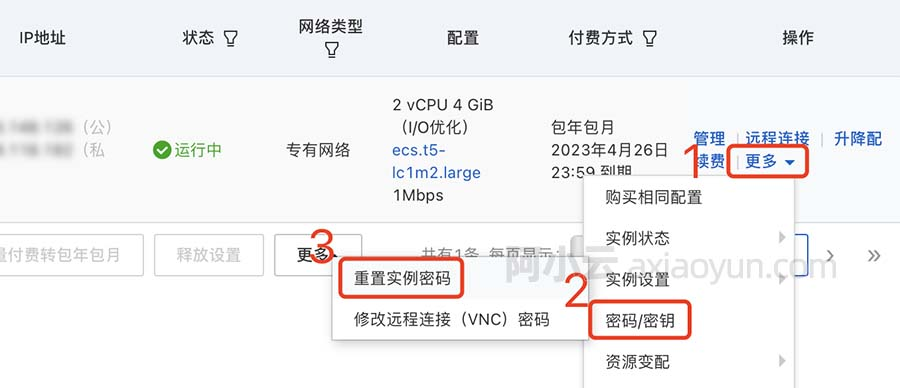22326 019260 016626 022472 019645 0windows server 2008阿里云ECS服务器安全设置

11997 0
400

0

JS零基础入门教程（上册）